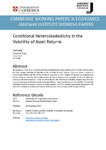## Conditional Heteroskedasticity in the Volatility of Asset Returns

Ding, Y.
##### DOIWorking Paper
##### Abstract

We propose a new class of conditional heteroskedasticity in the volatility (CHV) models which allows for time-varying volatility of volatility in the volatility of asset returns. This class nests a variety of GARCH-type models and the SHARV model of Ding (2021). CH-V models can be seen as a special case of the stochastic volatility of volatility model. We then introduce two examples of CH-V in which we specify a GJR-GARCH and an E-GARCH processes for the volatility of volatility, respectively. We also show a novel way of introducing the leverage effect of negative returns on the volatility through the volatility of volatility process. Empirical study confirms that CH-V models have better goodness-of-fit and out-of-sample volatility and Value-at-Risk forecasts than common GARCH-type models.

2021-11-08
##### Keywords
GARCH, SHARV, volatility, volatility of volatility, forecasting
##### Publisher
Faculty of Economics, University of Cambridge